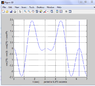# Combining Cos(t)'s from (sin2t + sin3t)/2*sint

• toneboy1

#### toneboy1

The question was given: x(t) = (sin2t + sin3t)/2*sint

determine the period. So I converted numerator and denominator into the exponential forms and ended up getting (after flipping, timesing and cancelling)
(exp(j3t)-exp(-j)-exp(j)+exp(-3j)+exp(j4)-exp(-2j)-exp(j2)+exp(-4j)) / 2

which in turn turns into

x(t) = cos(3t) - cos(t) - cos(2t) + cos(4t) if I'm not mistaken

so is there a way of simplifying this into maybe one Cos or something so I can find the period?

Or have I done this question the wrong way?

Thanks heaps!

use sin(2t)=2sin(t)cos(t). then write sin(3t)=sin(2t+t) and expand it. next use
$\cos(2t) = 2 \cos^{2}t - 1$. you then end up with
$$x(t) = \cos t + 2 \cos^{2}t - \frac{1}{2}$$

...then write sin(3t)=sin(2t+t) and

That is very clever.

So I got to:

(2.sin(t).cos(t) + sin(2t).cos(t)+cos(2t).sin(2t)) / 2sin(t)

[2.sin(t).cos(t)+2sin(t).cos(t).cos(t)+(cos^2(t)-sin^2(t))*(2.sin(t).cos(t)) ]
/ 2sint

(then) although I have ended up with cost+cos^2 t + cos^3 t - sin^2(t).cost

Have I done something wrong or am I not finished simplifying it? (I can't think of what else to do).

Thanks

Last edited:
The question was given: x(t) = (sin2t + sin3t)/2*sint

determine the period. So I converted numerator and denominator into the exponential forms and ended up getting (after flipping, timesing and cancelling)
(exp(j3t)-exp(-j)-exp(j)+exp(-3j)+exp(j4)-exp(-2j)-exp(j2)+exp(-4j)) / 2

which in turn turns into

x(t) = cos(3t) - cos(t) - cos(2t) + cos(4t) if I'm not mistaken

so is there a way of simplifying this into maybe one Cos or something so I can find the period?

Or have I done this question the wrong way?

Thanks heaps!
Once you have a sum of cosines, the period is the lowest common multiple of the periods of the terms.

That is very clever.

So I got to:

(2.sin(t).cos(t) + sin(2t).cos(t)+cos(2t).sin(2t)) / 2sin(t)

Have I done something wrong or am I not finished simplifying it? (I can't think of what else to do).

Thanks

sin(t+2t) = sin(t)cos(2t)+sin(2t)cos(t)

Once you have a sum of cosines, the period is the lowest common multiple of the periods of the terms.

What about if the Cosine is negative? Anyway so that would mean from the answer in my original post the period was 't'?

Thanks

sin(t+2t) = sin(t)cos(2t)+sin(2t)cos(t)

Isn't that what I did?

What about if the Cosine is negative? Anyway so that would mean from the answer in my original post the period was 't'?

Thanks
-cos(x)=cos(x+π)
That component will have a phase of π, in respect to the other components. Does not change the period, though.

-cos(x)=cos(x+π)
That component will have a phase of π, in respect to the other components. Does not change the period, though.

Ah, I see. So it was '1'. When I plotted the sum of: x(t) = cos(3t) - cos(t) - cos(2t) + cos(4t) in MATLAB it generated a period of 6.275 or something, how can this be?

Observe the picture attached

#### Attachments

•PF.png
12.8 KB · Views: 726
What would you expect? What is the period of cos(t)?

What would you expect? What is the period of cos(t)?

so from the result I got, how was 2π≈6.28?

so from the result I got, how was 2π≈6.28?

I am not sure what are you asking. The value of 2π is not a consequence of your result but the other way around.
π or "PI" is 3.1415...
so 2π= 6.28...

Now the period of a function like sin(x) or cos (x) is 2π. This means that sin(x+2π) = sin(x).
In these expressions x is a non-dimensional variable.

You can also write sin(x+360) = sin(x) but then your x should be in hexadecimal degrees too.

I am not sure what are you asking. The value of 2π is not a consequence of your result but the other way around.
π or "PI" is 3.1415...
so 2π= 6.28...

Now the period of a function like sin(x) or cos (x) is 2π. This means that sin(x+2π) = sin(x).
In these expressions x is a non-dimensional variable.

You can also write sin(x+360) = sin(x) but then your x should be in hexadecimal degrees too.

AH! Because I was plotting it like it was in Time (seconds)

Playing Devil's advocate, would it be fair to make 't' as seconds and plot the thing and say it took 6.28 seconds for a period?

In physics we usually make the arguments of the functions non-dimensional.
A physical quantity periodic in time will be represented by something like
sin(2∏t/T) so t is in seconds and T is the "physical" period, in seconds.
This has the property

sin[2∏(t+T)/T)]=sin(2∏t/T) so the period is T.

In physics we usually make the arguments of the functions non-dimensional.
A physical quantity periodic in time will be represented by something like
sin(2∏t/T) so t is in seconds and T is the "physical" period, in seconds.
This has the property

sin[2∏(t+T)/T)]=sin(2∏t/T) so the period is T.

what do you mean by 'non-dimensional' exactly?

Could I plead the case that since that T is 2π, it is 6.28 seconds?

How did the 'sin[2∏(t+T)/T)]' come about?

Isn't that what I did?

No, look again at your calculations. you put

$$\sin (2t) = \sin (2t) \cos (t) + \cos (2t) \sin (2t)$$

which is wrong.

No, look again at your calculations. you put

$$\sin (2t) = \sin (2t) \cos (t) + \cos (2t) \sin (2t)$$

which is wrong.

You're right, thanks, would you be happy with:

(2.sin(t).cos(t) + sin(2t).cos(t)+cos(2t).sin(t)) / 2sin(t)

[2.sin(t).cos(t)+2sin(t).cos(t).cos(t)+(cos^2(t)-sin^2(t))*sin(t)) ]
/ 2sint

∴ 2cost + 2cos^2(t) +Cos^2(t)-(1-cos^2(t)) / 2

∴ 2Cos^2(t) + Cos(t) - 1/2

which is about as simple as you can get, with a period 2π?

Thanks